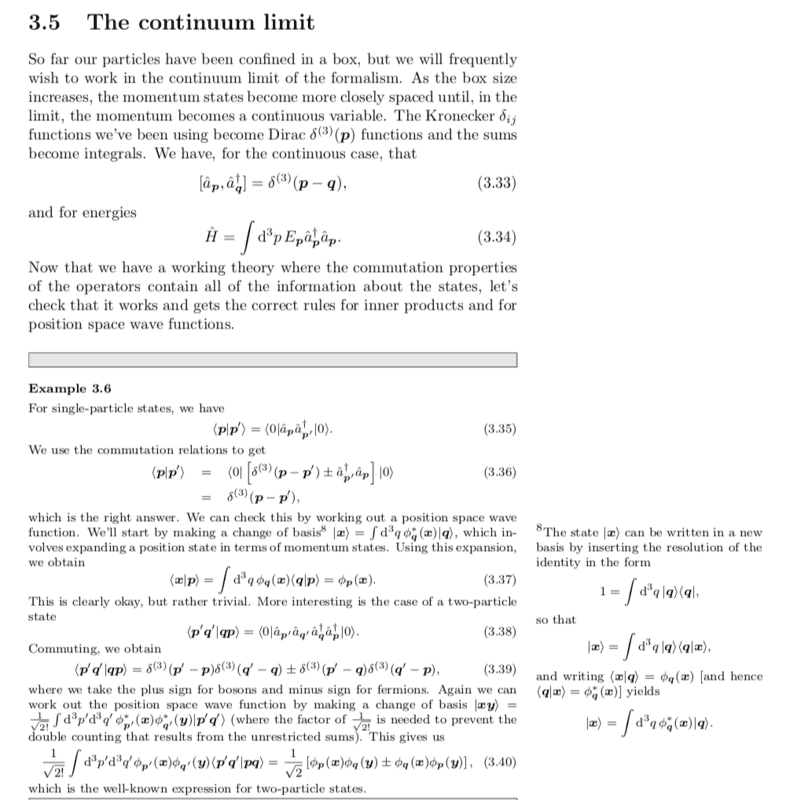# Need help regarding the derivation of a 2-particle wavefunction

Homework Statement:
From Blundell's QFT
Relevant Equations:
Fourier transforms
I have an issue trying to understand the derivation of equation 3.40 (screenshot attached) of Blundell's QFT book. Here's my attempt.

##| x,y \rangle = |x\rangle \otimes |y\rangle = \Big( \int dp' \phi_{p'}(x)|p'\rangle \Big) \otimes \Big( \int dq' \phi_{q'}(y)|q'\rangle \Big)## which gives ##\int dp' \int dq' \phi_{p'}(x) \phi_{q'}(y) |p',q'\rangle##.

I can't seem to understand the argument by Blundell that "the factor of ##1/\sqrt{2!}## is needed to prevent double counting that results from unrestricted sums". Why does the factor of ##1/\sqrt{2!}## not "pop out" on its own? Aren't fourier transforms supposed to preserve normalisation?

Cheers.## Answers and Replies

could someone assist? Many thanks

TSny
Homework Helper
Gold Member
Since no one has responded yet, I will try to help a little. I am not very familiar with this topic.

##| x,y \rangle = |x\rangle \otimes |y\rangle = \Big( \int dp' \phi_{p'}(x)|p'\rangle \Big) \otimes \Big( \int dq' \phi_{q'}(y)|q'\rangle \Big)## which gives ##\int dp' \int dq' \phi_{p'}(x) \phi_{q'}(y) |p',q'\rangle##.

You assumed here that ##|x,y \rangle = |x\rangle \otimes |y\rangle ## and ##|q,p \rangle = |p \rangle \otimes |q\rangle ##. But I don't think that's right. ##| x,y \rangle ## and ##| q,p \rangle## are two-particle states that are already symmetrized or antisymmetrized for bosons or fermions.

For example, the definition of ##| p,q \rangle## is ##| p,q \rangle = \hat a_p ^\dagger \hat a_q^ \dagger | 0 \rangle##. Using the commutation relations for the operators, you see that ##| p,q \rangle = \pm | q, p \rangle##, (+ for bosons, - for fermions). But, ##|p \rangle \otimes |q\rangle\neq \pm |q \rangle \otimes |p\rangle##.

I can't seem to understand the argument by Blundell that "the factor of ##1/\sqrt{2!}## is needed to prevent double counting that results from unrestricted sums". Why does the factor of ##1/\sqrt{2!}## not "pop out" on its own? Aren't fourier transforms supposed to preserve normalisation?
Here, I don't think I can help much. As I see it, the factor of ##1/\sqrt{2!}## is part of the definition of ##|x,y \rangle##. I'm not sure of the interpretation of Blundell's remark about preventing double counting. But, with the factor of ##1/\sqrt{2!}##, you can see that you get the properly normalized result in Blundell's (3.40).

•WWCY
Hi, thanks for the reply!

I completely forgot about the part regarding anti-symmetry/symmetric states.

However, would it make any sense if I performed the fourier transforms on this (symmetric) state? ##|x,y\rangle = \frac{1}{\sqrt{2}} ( |x \rangle |y\rangle + |y \rangle |x\rangle )##

TSny
Homework Helper
Gold Member
Hi, thanks for the reply!

I completely forgot about the part regarding anti-symmetry/symmetric states.

However, would it make any sense if I performed the fourier transforms on this (symmetric) state? ##|x,y\rangle = \frac{1}{\sqrt{2}} ( |x \rangle |y\rangle + |y \rangle |x\rangle )##

If you take ##|p, q \rangle## as corresponding to ##\frac{1}{\sqrt{2}}( |p \rangle |q \rangle + |q \rangle ||p \rangle)## and ##|x, y \rangle## as corresponding to ##\frac{1}{\sqrt{2}} (|x \rangle |y \rangle + |y \rangle |x \rangle )##, then ## \langle y, x | p, q \rangle## will not yield the factor of ##\frac{1}{\sqrt{2}}## in Blundell's (3.40). If you want to get that factor, it appears that you would have to take ##|x,y\rangle## as corresponding to ## \frac{1}{2} ( |x \rangle |y\rangle + |y \rangle |x\rangle )## which has an overall factor of ##\frac{1}{2}## instead of ##\frac{1}{\sqrt{2}}##. This corresponds to the extra factor of ##\frac{1}{\sqrt{2!}}## that Blundell includes in his definition of the change of basis from the basis ##|p, q \rangle ## to the basis ##|x, y \rangle##.